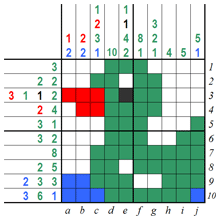﻿ Pic-a-Pix techniques
 Log inBecome a member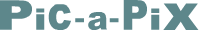# Pic-a-Pix techniques

Pic-a-Pix puzzles consist of a blank grid with clues on the left of every row and on the top of every column. The object is to reveal a hidden picture by painting blocks according to the clues. Each clue shows the length and the color of a block in the corresponding row or column, the blocks are organized in the same sequence as the clues, and there must be a space of at least one empty square between adjacent blocks. In the case of Color Pic-a-Pix it is allowed to have no empty squares between adjacent blocks if the adjacent blocks are of different colors.

For more Pic-a-Pix techniques see Pic-a-Pix tutorial & walkthroughs: A beginner’s guide to solving picture forming logic puzzles by Marilyn Dorant.

# B&W Pic-a-Pix step by step solution

B&W Pic-a-Pix puzzles are solved by selecting a row or column, one at a time, analyzing the clues and working out which ways the blocks can fit in. Using logic you will notice situations where some squares must be painted while others must remain empty. It is just as important to mark empty squares, which can be done with an X or a dot, as it is to paint squares which must be black. The best way to learn how to solve B&W Pic-a-Pix puzzles is to see a puzzle solved step by step from beginning to end.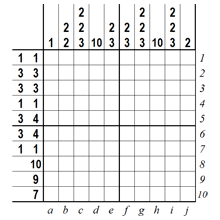## Step 1

Solving B&W Pic-a-Pix always starts with the longest blocks. In very easy puzzles there are sometimes blocks which span the entire grid and can be painted immediately as we see in row 8 and columns d and h below. It is recommended to check off solved clues to indicate they are no longer needed and to avoid confusions later on.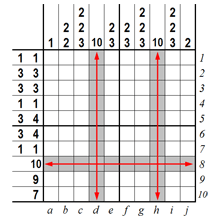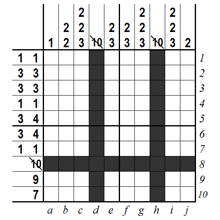## Step 2

The next step is solving rows and columns containing a single block larger than half of the grid. Let’s look at row 9 which contains a single block 9 squares long. No matter how we place this block to the right or to the left as indicated by the two red arrows there will always be an overlapping area of eight squares shown in gray below. We can therefore paint these squares in black. The same technique is used for row 10 which contains a single block 7 squares long.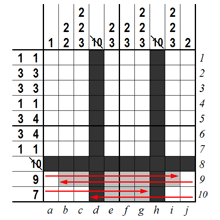## Step 3

Now let’s scan the puzzle vertically using previously painted squares as clues. Column a contains one square which was painted in the previous steps. This means the clue 1 is accounted for and we can mark all remaining squares with X to indicate they are empty. Column b contains two blocks of 2 squares each and the bottom block is already painted. We can therefore mark with X the squares immediately above and below to indicate they must remain empty. Columns e, f and g are solved in a similar way except only squares above the blocks are marked with X. Column j contains a single block of 2 squares, and one of these squares is already painted. Although we don’t know which adjacent square should be painted we can still mark most of the remaining squares with X since we know they are going to be empty.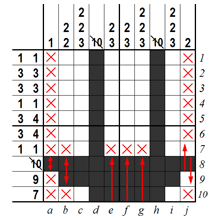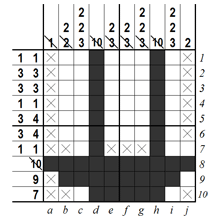## Step 4

We now go back to horizontal scanning where we see rows 1, 4 and 7 are fully accounted for. This means we can mark all remaining squares with X since they must obviously remain empty. Row 9 contains a single block of 9 squares, 8 of which are already painted so we can paint the last empty square as well.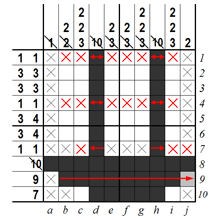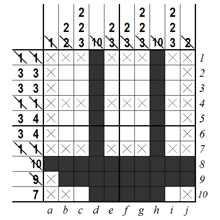## Step 5

Looking at column c it is clear that there is only one way to complete the block of 3 and paint the two remaining blocks of 2. Column i is solved the same way while column g is solved by painting the two remaining blocks of 2.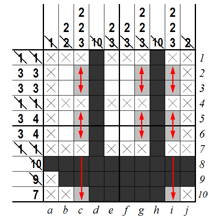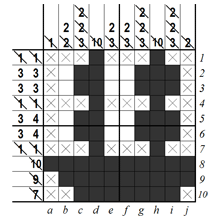## Step 6

We are now almost at the end. Rows 5 and 6 can now be completed having only one way to fit the blocks of 3 and 4. Row 2 contains two blocks of 3, one of which is completed. We can therefore mark X on the left of the block to indicate there must be a space. Row 3 is solved in the same way.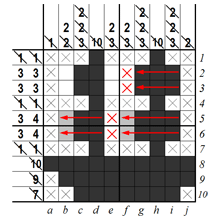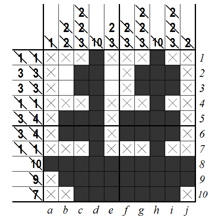## Step 7

In column b the two blocks of 2 are accounted for which means the remaining squares must marked with X to show they are empty. Column e has the opposite situation where one block of 2 squares is missing and there is only one way to fit it in. The last two squares are therefore painted and the puzzle is done.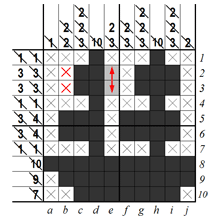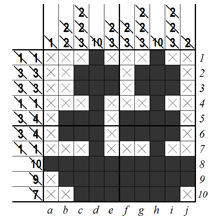## Step 8

The B&W Pic-a-Pix puzzle is now complete, revealing the picture of a sailboat.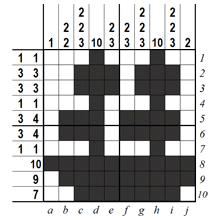# Color Pic-a-Pix step by step solution

Color Pic-a-Pix puzzles are solved the same way as B&W Pic-a-Pix with two additional rules: blocks are painted according to colors of the clues and it is allowed to have no empty squares between adjacent blocks if the blocks are of different colors. The best way to learn how to solve Color Pic-a-Pix puzzles is to see a puzzle solved step by step from beginning to end.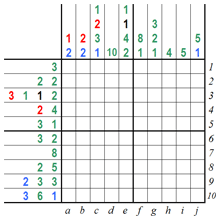## Step 1

Solving Color Pic-a-Pix always starts with the longest blocks. In very easy puzzles there are sometimes blocks which span the entire grid and can be painted immediately as shown in column d below. Another very easy situation is when two or more blocks and the spaces between them (if any) fit the grid exactly, as shown in column f. It is recommended to check off solved clues to indicate they are no longer needed and to avoid confusions later on.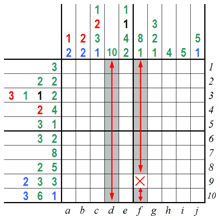## Step 2

Row 10 below is another very easy situation because the length of the three blocks adds up to 10 which is the exact width of the grid. Having covered the very easy steps we now search for rows and columns containing a single block larger than half of the grid. Let’s look at row 7 which contains a single block 8 squares long. No matter how we place this block to the right or to the left as indicated by the two red arrows, there will always be an overlapping area of six squares shown in gray below. We can therefore paint these squares in green according to the color of the clue.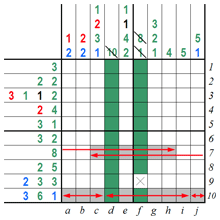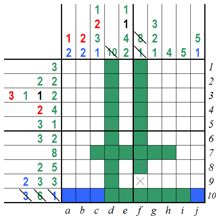## Step 3

Now let’s scan the puzzle vertically using previously painted squares as clues. Column a, as well as column b, contain a block of 2 blue squares one of which is already painted. Since the second blue square can only be the one above it is painted blue as well. Note that although the blocks of 2 blue squares have been solved we can’t mark an X above because the next block is red and there may be no space in between. Columns e, g, h and i are solved in the same way except an X is used in columns e and g since next block above is the same color and the remainder of columns h and i are marked with X since all remaining squares are empty.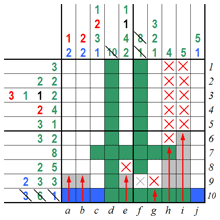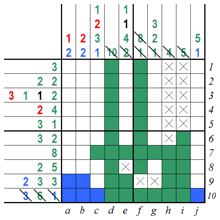## Step 4

Thanks to the squares painted in the previous steps we now see there is only one way to solve the green blocks in rows 1, 6, 8 and 9. All blocks in these rows can now be painted, empty squares marked with X, and clues checked off to indicate they are no longer needed.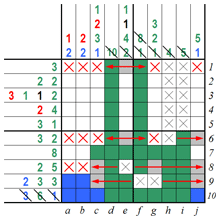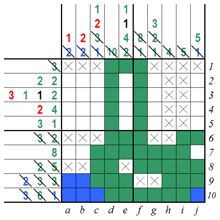## Step 5

Column e contains two green squares which are adjacent to an X so there is only one way to paint the remaining two squares. However, we cannot mark X at the top since the next block above is black. In column g we need to fit a green block of 3 in a space of 4 squares. No matter how we place this block to the top or to the bottom as indicated by the two red arrows, there will always be an overlapping area of two squares shown in gray below. We can therefore paint these squares in green according to the color of the clue. And finally, column j can be completed since it has only one solution.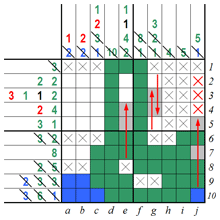## Step 6

We are now very close to the end of this puzzle. Similar to Step 4, we see there is only one way to solve the remaining blocks in rows 2, 3, 5 and 7. All blocks in these rows can now be painted according to the respective clue colors, empty squares marked with X, and clues checked off to indicate they are no longer needed.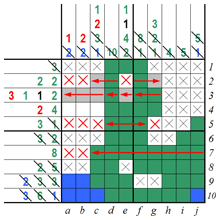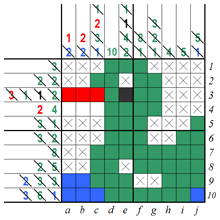## Step 7

In column a the red block of 1 square is accounted for which means the remaining square must marked with X to show it is empty. Columns b and c have the opposite situation where the remaining squares are painted in red and the puzzle is done.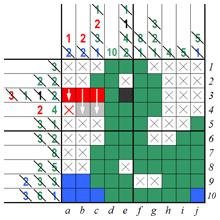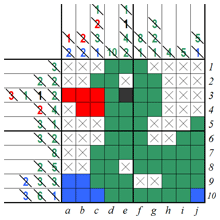## Step 8

The Color Pic-a-Pix puzzle is now complete, revealing the picture of a duck.